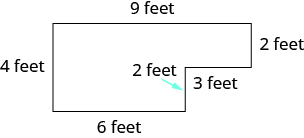Learning Outcomes

• Translate word phrases into mathematical notation representing addition
• Solve application problems by translating word phrases into mathematical expressions

Translate Word Phrases to Math Notation

We’ve seen a few problems where we translate math notation into words. Now we’ll reverse the process. We’ll translate word phrases into math notation. Some of the word phrases that indicate addition are listed in the table below.

Operation Words Example Expression

sum

increased by

more than

total of

$1$ plus $2$

the sum of $3$ and $4$

$5$ increased by $6$

$8$ more than $7$

the total of $9$ and $5$

$6$ added to $4$

$1+2$

$3+4$

$5+6$

$7+8$

$9+5$

$4+6$

example

Translate and simplify: the sum of $19$ and $23$.

Solution
The word sum tells us to add. The words of $19$ and $23$ tell us the addends.

 The sum of $19$ and $23$ Translate. $19+23$ Add. $42$ The sum of $19$ and $23$ is $42$.

example

Translate and simplify: $28$ increased by $31$.

try it

Translate and simplify: $29$ increased by $76$.

Translate and simplify: $37$ increased by $69$.

In the video below we present more examples of how to translate from words to expressions.

Now that we have practiced adding whole numbers, let’s use what we’ve learned to solve real-world problems. We’ll start by outlining a plan. First, we need to read the problem to determine what we are looking for. Then we write a word phrase that gives the information to find it. Next we translate the word phrase into math notation and then simplify. Finally, we write a sentence to answer the question.

example

Hao earned grades of $87,93,68,95,\text{and }89$ on the five tests of the semester. What is the total number of points he earned on the five tests?

try it

Some application problems involve shapes. For example, a person might need to know the distance around a garden to put up a fence or around a picture to frame it. The perimeter is the distance around a geometric figure. The perimeter of a figure is the sum of the lengths of its sides.

example

Find the perimeter of the patio shown.try it

The video below helps with finding the perimeter of an L-shaped Polygon involving hole numbers.